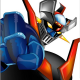Skip to content# Implementing the Strategy Pattern in ColdFusion

In spirit of the amazing book Head First Design Patterns, I wanted to put together a ColdFusion example that depicts the Strategy pattern. Essentially, the Strategy Pattern lets you group related algorithms together so that an object is able to select which algorithm to run at runtime.

So let’s say for example you had a parent class that has two properties and two methods. You now create a subclass that inherits the parent class, meaning that it will gobble up all the properties and methods (whether you like it or not) from the parent class. You can’t choose, for example, what methods you subclass needs, even if some methods don’t make sense for your subclass. It’s an all-or-nothing solution. Yes, you can override, but what if there were 10 methods to override? Also, what if you had to create other types of subclasses – you’ll have to override those as well. Things can get a little sloppy at the end. That’s where the strategy pattern comes in.

With this pattern, you first think about related methods and algorithms. (One method can have various algorithms; different implementations for doing the same thing.) For this blog post’s example, we’ll think of different ways a SuperHero can punch. To keep things simple, let’s give him two ways (two different algorithms for punching) he can punch. He can punch normally, or he can punch you, which freezes you as well. Let’s UML this to make things clearer.

First what the lines mean:Here’s the UML diagram for our SuperHero scenerio:From the diagram, you can see that the SuperHero abstract class is able to choose a punch set of algorithms (a strategy). We also see that Punch and PunchFreeze are implementation classes (classes that serve to implement an interface). Both of them have a punch() method that return void (nothing) – in this example, they’ll do stuff, and not return anything.

To make things a little more interesting, and to follow closer the example in the Strategy Pattern chapter of the book, we’re going to also create a subclass called Freezer that inherits the SuperHero class. Also, we’ll create another strategy for kicking. Here’s what the UML for that looks like:Here’s the CF code:

SuperHero.cfc

```<cfcomponent>

<!--- This is our abstract class. Responsibility to implement is delegated to --->
<!--- classes that implement interfaces. --->

<cffunction name="init" access="public" returntype="SuperHero">
<!--- Some other init code goes here.  --->
<cfargument name="name" type="string">
<cfargument name="gender" type="string">
<cfset this.name = arguments.name />
<cfset this.gender = arguments.gender />
<cfreturn this />
</cffunction>

<cffunction name="setPunchAlgorithm" access="public">
<!--- The next two lines are key. It's where you set the implementation --->
<!--- of punch from an object that's being passed in. --->
<cfargument name="PunchAlgorithm" type="IPunchAlgorithm" required="true" />
<cfset this.punchAction = PunchAlgorithm.punch />
</cffunction>

<cffunction name="setKickAlgorithm" access="public">
<cfargument name="KickAlgorithm" type="IKickAlgorithm" required="true" />
<cfset this.kickAction = KickAlgorithm.kick />
</cffunction>

<!--- This function will be overridden.  --->
<cffunction name="energyProject" access="public" returntype="SuperHero" >
You have been pointed at by a weak flash light.
<cfreturn this />
</cffunction>

</cfcomponent>
```

IPunchAlgorithm.cfc

```<cfinterface>

<cffunction name="punch" access="public" />

</cfinterface>
```

Punch.cfc

```<cfcomponent implements="IPunchAlgorithm">

<cffunction name="punch" access="public">
You have been punched normally. Ouch.
</cffunction>

</cfcomponent>
```

PunchFreeze.cfc

```<cfcomponent implements="IPunchAlgorithm">

<cffunction name="punch" access="public">
You have been punched and are now frozen, stuck. Good luck thawing!
</cffunction>

</cfcomponent>
```

IKickAlgorithm.cfc

```<cfinterface>

<cffunction name="kick" access="public" />

</cfinterface>
```

Kick.cfc

```<cfcomponent implements="IKickAlgorithm">

<cffunction name="kick" access="public">
You have been kicked in the gut. Yummy.
</cffunction>

</cfcomponent>
```

Freezer.cfc

```<cfcomponent extends="SuperHero">

<!--- We are overriding the energyProject from Freezer's parent class.  --->
<cffunction name="energyProject">
You have been snowed on.
</cffunction>

</cfcomponent>
```

Now let’s actually use these the pattern:

run.cfm

```<!--- Create context object.  --->
<cfset IceMan = CreateObject( "component", "SuperHero" ).init( Name = "Iceman", Gender = "Male" ) />

<!--- Create a strategy #1 for punching.  --->
<cfset PunchStrategy_1 = CreateObject( "component", "Punch" ) />

<!--- Create a different strategy for punching.  --->
<cfset PunchStrategy_2 = CreateObject( "component", "PunchFreeze" ) />

<!--- Tell the IceMan object that you'll be using the Punch Strategy #2, NOT #1.  --->
<cfset IceMan.setPunchAlgorithm( PunchStrategy_2 ) />

<!--- Now let's create a kicking strategy... --->
<cfset KickStrategy = CreateObject( "component", "Kick" ) />

<!--- ... and now let's tell the IceMan object that you'll be using the Kick Strategy.  --->
<cfset IceMan.setKickAlgorithm( KickStrategy ) />

<!--- Now let's see some action!  --->

<!--- Punch, using the strategy chosen! --->
<cfset IceMan.punchAction() />

<!--- Punch, using the strategy chosen! --->
<cfset IceMan.kickAction() />

<!--- Let's create another SuperHero object. --->
<cfset Frosty = CreateObject( "component", "SuperHero" ).init( Name = "Frosty the Snowman", Gender = "Unknown" ) />

<!--- Let's inspect the objects --->
<p>Notice that both objects have different number of methods - only the methods they need. </p>
<cfdump var="#IceMan#" /><hr />
<cfdump var="#Frosty#" /><hr />

<!--- Let's create another SuperHero object. --->
<cfset Frostman = CreateObject( "component", "Freezer" ).init( Name = "Calvin Hobbes", Gender = "Male" ) />
<cfset Frostman.energyProject() />
```

You can download all the CF code here with the original Visio diagram source.

CategoriesBlog owner.